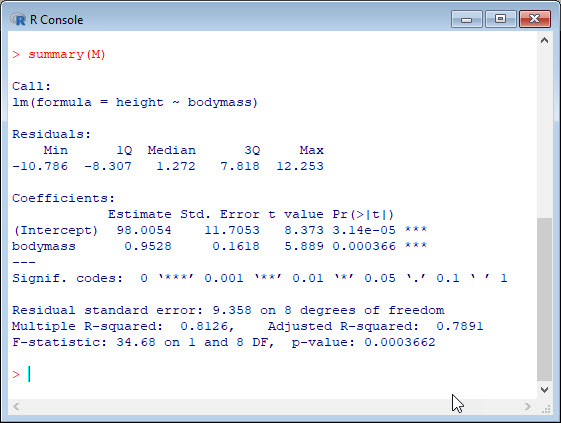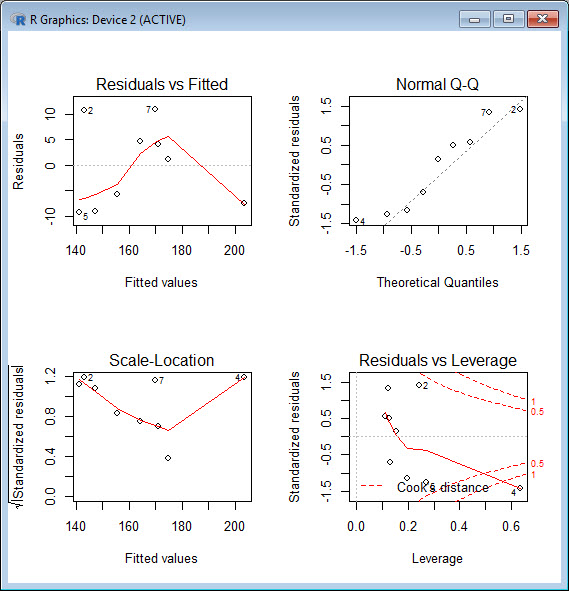Last time we created two variables and used the `lm()` command to perform a least squares regression on them, and diagnosing our regression using the `plot()` command. Here are the data again.

```height = c(176, 154, 138, 196, 132, 176, 181, 169, 150, 175) bodymass = c(82, 49, 53, 112, 47, 69, 77, 71, 62, 78)```

Just as we did last time, we perform the regression using `lm()`. This time we store it as an object `M`. Indeed – R allows you to do that!

`M <- lm(height ~ bodymass)`

Now we use the `summary()` command to obtain useful information about our regression:

`summary(M)`Our model p-value is very significant (approximately 0.0004) and we have very good explanatory power (over 81% of the variability in height is explained by body mass).

We saw in the previous blog that points 2, 4, 5 and 6 have great influence on the model. Now we see how to re-fit our model while omitting one datum. Let’s omit point 6. Note the syntax we use to do so, involving the `subset()` command inside the `lm()` command and omitting the point using the syntax `!=` which stands for “not equal to”. The syntax instructs R to fit a linear model on a subset of the data in which all points are included except the sixth point.

`M2 <- lm(height ~ bodymass, subset=(1:length(height)!=6))`
`summary(M2)`Because we have omitted one observation, we have lost one degree of freedom (from 8 to 7) but our model has greater explanatory power (i.e. the Multiple R-Squared has increased from 0.81 to 0.85). From that perspective, our model has improved, but of course, point 6 may well be a valid observation, and perhaps should be retained. Whether you omit or retain such data is a matter of judgement.

Our diagnostic plots were as follows:When comparing them with the diagnostic plots in previous blog we can see that there are no significant changes in these plots. In other words, omitting point 6 didn’t improve quality of the regression.

David

#### Annex: R codes used

```# Create two variables.
height = c(176, 154, 138, 196, 132, 176, 181, 169, 150, 175)
bodymass = c(82, 49, 53, 112, 47, 69, 77, 71, 62, 78)

# Store the regression model as an object.
M <- lm(height ~ bodymass)

# Obtain useful information about regression.
summary(M)

# Store regression model as object after omitting point 6.
M2 <- lm(height ~ bodymass, subset=(1:length(height)!=6))

# Obtain useful information about new regression.
summary(M2)

# Create a plotting environment of two rows and two columns and plot the model.
par(mfrow = c(2,2))
plot(M2)
```